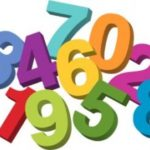# Real Valued Function (Real Function)

Simply stated, a real valued function has real numbers for values.

Let’s suppose you have two sets of real numbers, X and Y. Using the definition of a function, we can define the real valued function as being a correspondance that assigns each input (x) in the set X to exactly one output (y) in the set Y.

Real valued functions for the basic of many natural phenomenon and are the types of functions usually dealt with in introductory calculus classes.

## What are Real Numbers?

Real numbers (or reals) are any numbers that can be represented on the real number line—the one you’re used to seeing in basic math:

The set of reals includes both the set of rational numbers (numbers that can be written as ratios or fractions) and the set of irrational numbers (numbers that can’t be written as fractions, like pi).

There are an infinite number of reals, and there is an infinite number of reals between any two reals. For example, between 1 and 2 there are 0.1, 0.21, 0.239, and so on.

All whole numbers (1, 2, 3, 4…) and integers (-2098, -2, 1, 2…) are also included in the set of reals.

The set of reals is denoted by the symbol ℝ .

## What Number is Not a Real Number?

Imaginary numbers of the from a + ib, with i= √(-1) and b ≠ 0, are not reals.

## Core Properties of Real Numbers.

Since every real number has its own place along the number line, reals can be ordered. Properties of reals include:

• The Distributive Property: a(b + c)= ab +ac for every a, b, and c in ℝ.
• The Commutative Property: ab = ba and a + b = b + a for a and b in ℝ.
• The Associative Property: (ab)c = a(bc) and (a + b) + c= a + (b + c) for all a, b, and c in ℝ
• The Closure Property: a + b, a – b, and ab are all reals if a and b are reals; that is to say, reals are closed under addition, subtraction, and multiplication.

## The Identity Properties of Reals

There is one multiplicative identity, 1, and one additive identity, 0, such that

1a = a and 0 + a = a
for every a in ℝ.The multiplicative identity tells us that if you multiply any number by one the result is the number itself, in other words, when you multiply a number by 1 it doesn’t change the number’s value. For example:

• 10 x 1 = 10
• 1 x 10 = 10

The theory behind the multiplicative identity property is the same as that behind multiplication in general; all multiplications break down to a series of additions. Multiplying any number by 1 is the same as adding that number to zero once.

## Multiplicative Inverse

The multiplicative inverse or reciprocal for a number x, denoted by 1/x or x-1, is a number that when multiplied by x gives us the multiplicative identity of 1. This concept is more easily understood using some examples.

## Examples: Finding the Multiplicative Inverse

1. What is the multiplicative inverse of 13?

Translated, the question is: which number when multiplied by 13 would give us 1 (the multiplicative identity)? We can use x for the unknown multiplicative inverse.

13(x) = 1
x = 1/13

1/13 is the answer. The multiplicative inverse of any number is that number as the denominator and 1 as the numerator. Multiplying 13 by 1/13 equals 1.

1. What is the multiplicative inverse of ¼?

Here we are dealing with a fraction so the process is different. We will again use x for our unknown multiplicative inverse.

1/4 (x) = 1
x = 1 / ¼
(1/1)/(¼) = (1/1)(4/1) = 4

Our answer is that 4 is the multiplicative inverse of ¼. The only difference from the example above is that when dividing fractions we flip the numerator and denominator of the second fraction and multiply.

## The Inverse Properties of Reals

Every real number a has a unique additive inverse –a such that a + (-a) = 0, with –a being a member of the set of real numbers. Every nonzero real number a also has a unique multiplicative inverse 1/a such that a(1/a) = 1, where 1/a is a real number.

## Real Valued Function of Two, Three Variables

A “function of two variables” or “function of three variables” is just a statement that tells you how many inputs you’re dealing with. As an example, let’s say you were measuring the temperature t in a greenhouse experiment. If you measured at the plant height (p) and ceiling (c), you would have a real valued function of two variables. It can be written (where ℝ is the notation for “the set of real numbers”) as:

T: ℝ2 : (c, p) ↦ T (c, p)

If you add a third location, say, door (d), then you have a real function of three variables:

T: ℝ3 : (c, d, p) ↦ T (c, d, p)

The notation “↦” means “maps to”. If you’re familiar with the concept of a function, this should make sense: the inputs of the function (on the left) are mapping to the outputs of the function on the right.

Related article: Mapping diagrams of functions.

## Real Valued Function: References

Larson, R. & Edwards, B. (2008). Calculus of a Single Variable. Cengage Learning.
Binegar, B. Real-valued functions. Retrieved November 24, 2019 from: https://math.okstate.edu/people/binegar/4013-U98/4013-l04.pdf
The Improving Mathematics Education in Schools (TIMES) Project (2011). Retrieved June 15, 2018 from: http://amsi.org.au/teacher_modules/Real_numbers.html
Schechter, E. What are the “real[s],” really? Retrieved June 15, 2018 from: https://math.vanderbilt.edu/schectex/courses/thereals/
Peters, C. (n.d.). The Real[s]. Retrieved June 15, 2018 from: https://www.math.uh.edu/~charles/Real-nos.pdf

CITE THIS AS:
Stephanie Glen. "Real Valued Function (Real Function)" From StatisticsHowTo.com: Elementary Statistics for the rest of us! https://www.statisticshowto.com/real-valued-function/